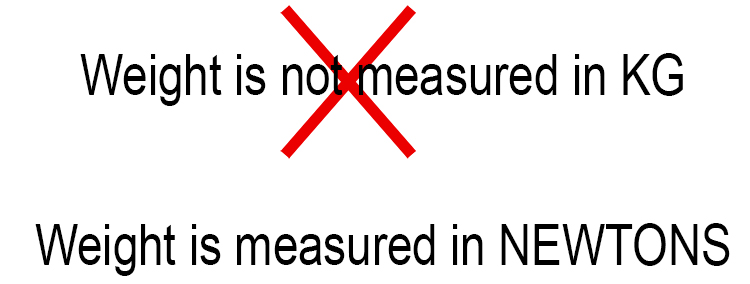# Weight is Not

Remember, in physics:

Weight is a force (force = m x a)

Weight = m x g

(Where gravity is the acceleration of the earths pull i.e. the force of gravity).